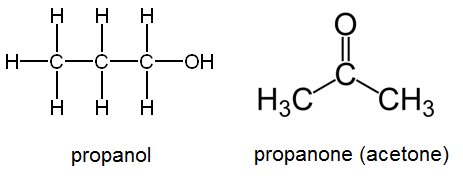# 25.17: Oxidation Reactions

Benzoic acid is widely used as a food preservative, either as the carboxylic acid or as the sodium benzoate salt. This compound is most effective when added to acidic foods such as fruit juices and soft drinks. The major industrial source of benzoic acid is the partial oxidation of toluene with oxygen. The process is inexpensive and environmentally benign.

## Oxidation Reactions

Oxidation can be defined as the addition of oxygen to a molecule, or the removal of hydrogen from a molecule. When an alkane is heated in the presence of an appropriate catalyst, it can be oxidized to the corresponding alkene in a reaction called a dehydrogenation reaction. Two hydrogen atoms are removed in the process. The alkene can be further oxidized to an alkyne by the removal of two more hydrogen atoms:

$\text{oxidation:} \: \: \ce{CH_3CH_3} \overset{\ce{-H_2}}{\rightarrow} \ce{CH_2=CH_2} \overset{\ce{-H_2}}{\rightarrow} \ce{CH \equiv CH}$

The reactions are reversible, and so an alkyne can be reduced first to an alkene, and then to an alkane:

$\text{reduction:} \: \: \ce{CH \equiv CH} \overset{\ce{_H_2}}{\rightarrow} \ce{CH_2=CH_2} \overset{\ce{+H_2}}{\rightarrow} \ce{CH_3CH_3}$

The alkane is the most reduced form of a hydrocarbon, while the alkyne is the most oxidized form.

Oxidation reactions in organic chemistry often involve the addition of oxygen to a compound, which changes the particular functional group of that compound. The following sequence shows how methane can be oxidized first to methanol, then to methanal, then to methanoic acid, and finally to carbon dioxide.

$\begin{array}{ccccccccc} \ce{CH_4} & \overset{\text{gain of oxygen}}{\longrightarrow} & \ce{CH_3OH} & \overset{\text{loss of hydrogen}}{\longrightarrow} & \ce{CH_2O} & \overset{\text{gain of oxygen}}{\longrightarrow} & \ce{HCOOH} & \overset{\text{loss of hydrogen}}{\longrightarrow} & \ce{CO_2} \\ \text{methane} & & \text{methanol} & & \text{methanal} & & \text{methanoic acid} & & \text{carbon dioxide} \end{array}$

Each step in the process is either a gain of oxygen or a loss of hydrogen. Each step also releases energy, which explains why the complete combustion of alkanes to carbon dioxide is an extremely exothermic reaction.

The oxidation of an alcohol can produce either an aldehyde or a ketone. Ethanol can be oxidized in the laboratory through a heating process combined with the addition of an oxidizing agent such as the dichromate ion, which catalyzes the reaction in an acidic solution. The reaction produces the aldehyde ethanal (acetaldehyde).

$\ce{CH_3CH_2OH} \underset{\ce{H^+}}{\overset{\ce{Cr_2O_7^{2-}}}{\rightarrow}} \ce{CH_3CHO}$When the alcohol to be oxidized is a secondary alcohol, the oxidation product is a ketone rather than an aldehyde. The oxidation of the simplest secondary alcohol, 2-propanol, yields propanone.

$\ce{CH_3CHOCH_3} \underset{\ce{H^+}}{\overset{\ce{Cr_2O_7^{2-}}}{\rightarrow}} \ce{CH_3COCH_3}$Tertiary alcohols cannot be oxidized in this way, because the carbon to which the hydroxyl group is attached does not have another hydrogen atom attached to it.

When a primary alcohol is oxidized to an aldehyde, the reaction is difficult to stop because the aldehyde is easily oxidized further to the corresponding carboxylic acid. The oxidation of ethanal produces ethanoic (acetic) acid.

$\ce{CH_3CHO} \underset{\ce{H^+}}{\overset{\ce{Cr_2O_7^{2-}}}{\rightarrow}} \ce{CH_3COOH}$

Ethanol-containing beverages, such as wine, are susceptible to such oxidation if kept for long periods of time after having been opened and exposed to the air. Wine that has become oxidized will have an unpleasant vinegary taste due to the production of acetic acid.

Unlike aldehydes, ketones are resistant to further oxidation because the carbonyl group is in the middle of the carbon chain, and so the ketone cannot be converted to a carboxylic acid.

## Summary

• Oxidation can be defined as the addition of oxygen to a molecule, or the removal of hydrogen from a molecule.
• Primary alcohols can be oxidized to aldehydes, and then further oxidized to carboxylic acids.
• When the alcohol to be oxidized is a secondary alcohol, the oxidation product is a ketone rather than an aldehyde.
• Tertiary alcohols cannot be oxidized.